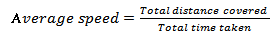# Very Short Question Answers: Motion & Time Notes | Study Science Class 7 - Class 7

## Class 7: Very Short Question Answers: Motion & Time Notes | Study Science Class 7 - Class 7

The document Very Short Question Answers: Motion & Time Notes | Study Science Class 7 - Class 7 is a part of the Class 7 Course Science Class 7.
All you need of Class 7 at this link: Class 7

Very Short Q&A:

Q1: The basic unit of speed is

(i) Km/ min

(ii) m/ min

(iii) Km/ sec

(iv) m/ sec

Ans : (iv) m/ sec

Q2: A faster moving object covers more distance in _________ time.

Ans : less

Q3: (Speed = Distance/ Time). True/ False

Ans : True

Q4: What is the SI unit of distance?

Ans : Meter

Q5: Name an object that shows oscillatory motion.

Ans : Pendulum

Q6: What is the motion of our hands while running?

Ans : oscillatory motion

Q7: What is the motion of a child in a merry-go-round?

Ans :

straight line motion

Q8: The time period of a given pendulum is not constant.True/ False

Ans : False

Q9: What is motion?

Ans : Motion is a change in the position of an object with time.

Q10: What is circular motion?

Ans : Motion of an object in a circular path is called circular motion e.g. Motion of the hands of a clock.

Q11: If the speed of an object keeps changing in equal interval of time, while moving along a straight line, its motion is said to be ___________________.

Ans : Non-uniform

Q12: The to and fro motion of an object from the position of rest is called a___________________.

Ans : Oscillatory motion.

Q13: One complete to and fro motion of a pendulum from rest position is called one______________.

Ans : One oscillation

Q14: Give an example of vibratory motion.

Ans : Simple Pendulum

Q15: Name the device on vehicles to track the distance covered.

Ans : An odometer

Q16: The basic unit of time is _________.

Ans : Second

Q17: Define average speed.

Ans : The average speed of a moving object is defined as the total distance covered by it divided by the total time taken.Q18: What do you mean by the statement; “car is moving with the speed of 50 Km per hour”?

Ans : Car is moving with the speed of 50 Km per hour it means it will cover a distance of 50 Km in one hour

Q19: Define uniform motion.

Ans : Objects that move in a straight line and maintain the same speed throughout the distance covered are said to be in uniform motion.

Q20: Define non- uniform motion.

Ans : Objects that move in straight line and whose speed varies are said to be in non-uniform motion

Q21: The metallic ball in pendulum is called __________ of the pendulum.

Ans : Bob

Q22: Give an example of oscillatory motion.

Ans : To and fro motion of a simple pendulum

Q23: The symbol of all units is written in _________________.

Ans : Singular

Q24: ________________motion may be along a straight line or along a curved path.

Ans : Translatory

Q25: Motion along a curved path is called _______________.

Ans : curvilinear motion

Q26: Name the device used to measure speed.

Ans : Speedometer

Q27: What type of graph is used to represent motion of an object?

Ans : distance-time graph

Q28: For a body in non-uniform motion, the graph is not a straight line. True/ False.

Ans : True

Q29: The working of a pendulum clock is based on the _____________  of its pendulum.

Ans : time period

Q30: Motion of the hammer of an electric bell is ___________________ motion.

Ans : Oscillatory motion

The document Very Short Question Answers: Motion & Time Notes | Study Science Class 7 - Class 7 is a part of the Class 7 Course Science Class 7.
All you need of Class 7 at this link: Class 7Use Code STAYHOME200 and get INR 200 additional OFF

## Science Class 7

161 videos|216 docs|54 tests

Track your progress, build streaks, highlight & save important lessons and more!

,

,

,

,

,

,

,

,

,

,

,

,

,

,

,

,

,

,

,

,

,

;